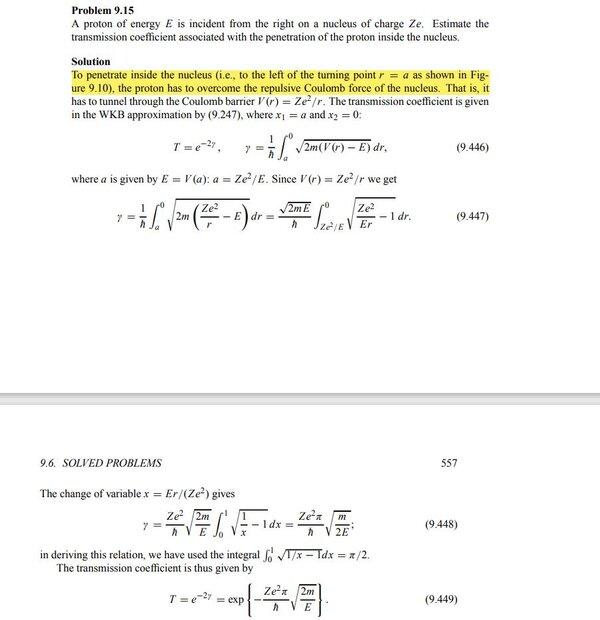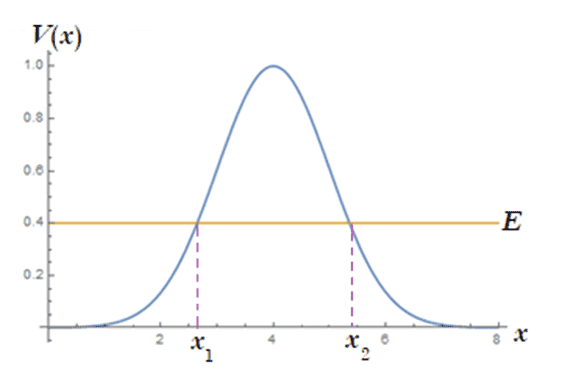# Limits of Integration in the Transmission Coefficient

• Samama Fahim
In summary, there appears to be a misprint in the limits of integration for equation 9.448 of the textbook. The limits should not be switched, as this would change the transmission coefficient. The substitution made in the following line also leads to a different result, but using the Beta function identity, the correct result can be obtained. f

#### Samama Fahim

Homework Statement
Why are the limits of integration swapped in the following equation without introducing a minus sign?
Relevant Equations
##\int_0^1\sqrt{\frac{1}{x}-1}dx##Initially '0' is the upper limit and ##a = \frac{Ze^2}{E}## is the lower limit. With change of variable ##x = \frac{Er}{Ze^2}##, for ##r=0##, ##x=0##, and for ##r=\frac{Ze^2}{E}##, ##x=1##, so 1 should be the lower limit. However, he takes 1 as the upper limit, and without a minus sign. Why is that?

(from Quantum Mechanics, Nouredine Zettili)

It appears to me that the error is in equation (9.446) of the text. ##\gamma## should be positive. But the way the limits are set up in the integral of (9.446), ##\gamma## will come out negative (since ##dr## in the integral will be negative). So, I think the limits should be switched in this integral with the lower limit being zero. The text refers back to (9.247). Does (9.247) agree with (9.446)?

•Delta2 and vela
It appears to me that the error is in equation (9.446) of the text. ##\gamma## should be positive. But the way the limits are set up in the integral of (9.446), ##\gamma## will come out negative (since ##dr## in the integral will be negative). So, I think the limits should be switched in this integral with the lower limit being zero. The text refers back to (9.247). Does (9.247) agree with (9.446)?
9.247 reads ##T \backsim e^{-2\gamma}##, ##\gamma = \frac{1}{\hbar}\int_{x_1}^{x_2}\sqrt{2m(V-E)} dx##

9.247 reads ##T \backsim e^{-2\gamma}##, ##\gamma = \frac{1}{\hbar}\int_{x_1}^{x_2}\sqrt{2m(V-E)} dx##
OK. This was probably discussed for the case where the particle tunnels from left to right through the barrier. That is, ##x_1## is the turning point on the left side and ##x_2## is the turning point on the right side. So, ##x_2 > x_1##.

The same formula (with no change in the integration limits for ##\gamma##) is valid in the WKB approximation if the particle tunnels from right to left through the barrier. The textbook appears to have made the mistake of assuming that the limits of integration should be switched in this case. You shouldn't switch the limits.

For example, suppose the potential barrier is symmetrically shaped, as shown.Clearly, the transmission coefficient ##T## for right-to-left tunneling is the same as for left-to-right tunneling. In both cases, the turning point on the left (##x_1##) should be the lower limit of integration in the expression for ##\gamma##. Switching the limits would change ##T##.

•Samama Fahim
I think there was a misprint in the limits of integration of equ. 9.448. You have
$$\frac{Ze^2\sqrt{2m}}{\hbar \sqrt{E}}\int_1^{0}\sqrt{\frac{1}{x}-1}dx$$
Make the substitution ##t=\frac{1}{x}-1## with ##dx=-\frac{dt}{(u+1)^2}## the integral becomes
$$I=-\frac{Ze^2\sqrt{2m}}{\hbar \sqrt{E}}\int_{\infty}^0 \frac{t^{\frac{1}{2}}}{(t+1)^2}dt$$
$$=\frac{Ze^2\sqrt{2m}}{\hbar \sqrt{E}}\int_{0}^{\infty} \frac{t^{\frac{1}{2}}}{(t+1)^2}dt$$
Using the formula for the Beta function
$$B(x,y)=\int_{0}^{\infty} \frac{t^{x-1}}{(t+1)^{x+y}}dt$$
and the Beta function in terms of Gamma functions,
$$B(x,y)=\frac{\Gamma (x) \Gamma (y)}{\Gamma (x +y)}$$
We find
$$I=\frac{Ze^2\sqrt{2m}}{\hbar \sqrt{E}}B(\frac{3}{2},\frac{1}{2})=\frac{\pi Ze^2\sqrt{m}}{\hbar \sqrt{2E}}$$
which is the obtained result in equ. 9.448.

I think there was a misprint in the limits of integration of equ. 9.448. You have
$$\frac{Ze^2\sqrt{2m}}{\hbar \sqrt{E}}\int_1^{0}\sqrt{\frac{1}{x}-1}dx$$
Make the substitution ##t=\frac{1}{x}-1## with ##dx=-\frac{dt}{(u+1)^2}## the integral becomes
$$I=-\frac{Ze^2\sqrt{2m}}{\hbar \sqrt{E}}\int_{\infty}^0 \frac{t^{\frac{1}{2}}}{(t+1)^2}dt$$

Wouldn't the limits of integration be switched in the last line above? The substitution ##t=\frac{1}{x}-1## gives ##t = 0## when ##x= 1##, and ##t = \infty## when ##x = 0##.

So, with the substitution ##t=\frac{1}{x}-1##, $$\int_1^0\sqrt{\frac{1}{x}-1}dx = -\int_0^\infty \frac{t^{\frac{1}{2}}}{(t+1)^2}dt$$